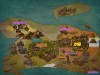# 星星灰暗着。# 星星灰暗着。

### FlashHu&oyiya的LCT题单

posted on 2018-12-09 10:13:56 | under Diary |

## $Chain$

### $[luoguP3613]$ 睡觉困难综合征（half half）

$lxl$ 好强啊。

$n\times k\times \log n\approx 100000\times 64\times 17=108800000$ ，加上 $LCT$ 的常数，惨痛。

### $[THUWC2017]$ 在美妙的数学王国中畅游（zero half）

$$e^{x}=\sum_{i=0}^{\infty}\frac{x^i}{i!}$$
$$\sin(x)=\sum_{i=0}^{\infty}(-1)^i\frac{x^{2i+1}}{(2i+1)!}$$

### $[luoguP1501]$ Tree II（half full）

$Mul$ 标记也要下放。

### $[bzoj2555]$ Substring（half half）

$\cdots\cdots$ 等等我都知道维护这个了为啥还要用 $LCT$ 啊？ $SAM$ 本身不就是一个增量构造吗 $\cdots\cdots$

## $MST$

### $[bzoj3514]$ Codechef MARCH14 GERALD07加强版（zero half）

$Rudy$ 的 $NOIp$ 模拟题有一个套路：一个环里的每条边都记一下这个环的最小编号，如果当前给定的某些边没有最小编号，那就不会成环。因为具体的题目描述我忘记了，所以只记得大概长这个样子

## $Subtree$

### $[bzoj3510]$ 首都（full half）

$UPD:$ 为什么网友们除了两个 $log$ 的启发式合并就是路径上二分啊？？？直接 $access$ 有错吗 $qwq$
$UPDD:$ 我知道了，必须要二分，因为 $access$ 统计的路径信息不完全。要在 $access$ 之后的 $splay$ 上二分，找到那个每个结点统计的在树上的实际子树和与 $n-$ 这个东西差最小的那个儿子就可以了。那这样的话感觉换根不太方便，可以考虑写启发式合并的做法。

### $[spoj16580]QTREE\ 7$ （zero half）

$QTREE\ 6$ 的加强版。但需要维护子树最大值，并资瓷删除。

## $Special$

### $[ZJOI2016]$ 大森林（half half）

ZJOI2016的大森林和小星星我都没想出来，要退役了啊

### $[ZJOI2018]$ 历史（half half）

$UPD:$ 果然，链做法和正解半点关系都没有。